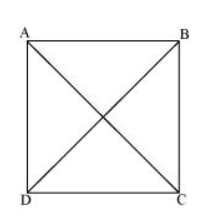# ABCD is a rectangle in which diagonal AC bisects ∠A as well as ∠C. Show that:<br/><br/>(i) ABCD is a square <br/><br/>(ii) diagonal BD bisects ∠B as well as ∠D.

Solution:(i) It is given that $\mathrm{ABCD}$ is a rectangle.

$\therefore \angle \mathrm{A}=\angle \mathrm{C}$

$\Rightarrow \frac{1}{2} \angle \mathrm{A}=\frac{1}{2} \angle \mathrm{C}$

$\Rightarrow \angle \mathrm{DAC}=\angle \mathrm{DCA} \quad($ AC bisects $\angle \mathrm{A}$ and $\angle \mathrm{C})$

$C D=D A$ (Sides opposite to equal angles are also equal)

However, $D A=B C$ and $A B=C D$ (Opposite sides of a rectangle are equal)

$\therefore \mathrm{AB}=\mathrm{BC}=\mathrm{CD}=\mathrm{DA}$

$A B C D$ is a rectangle and all of its sides are equal.

Hence, $A B C D$ is a square.

(ii) Let us join BD.

In $\triangle \mathrm{BCD}$

$B C=C D$ (Sides of a square are equal to each other)

$\angle C D B=\angle C B D$ (Angles opposite to equal sides are equal)

However, $\angle C D B=\angle A B D$ (Alternate interior angles for $A B \| C D$ )

$\therefore \angle C B D=\angle A B D$

$\Rightarrow \mathrm{BD}$ bisects $\angle \mathrm{B}$.

Also, $\angle C B D=\angle A D B$ (Alternate interior angles for $B C \| A D$ )

$\Rightarrow \angle C D B=\angle A B D$

$\therefore \mathrm{BD}$ bisects $\angle \mathrm{D}$.